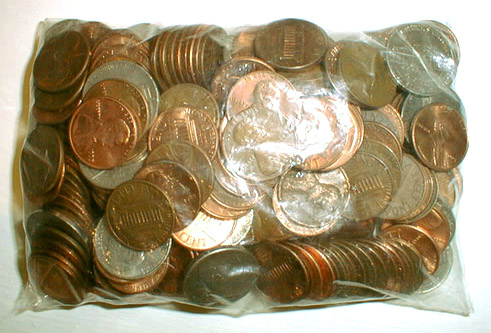to japanese | index | next

US\$2.84 Which Consists of 220 Coins (Money Amount No. 26)

The U. S. coins, a plastic bag
2002

cf. 220 and 284 are the smallest amicable pair which Pythagoras et al. found in about 500 B. C.
(Two numbers are called amicable if each equals to the sum of the divisors of the other.)
Divisors of 220: 1 + 2 + 4 + 5 + 10 + 11 + 20 + 22 + 44 + 55 + 110 = 284
Divisors of 284: 1 + 2 + 4 + 71 + 142 = 220